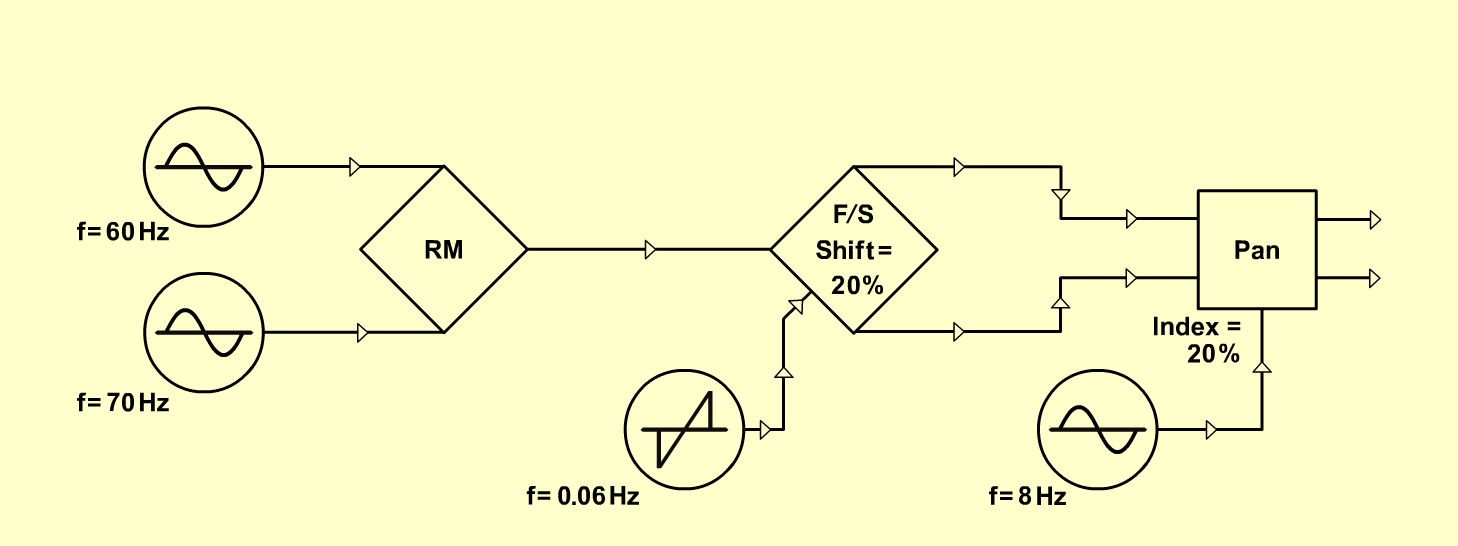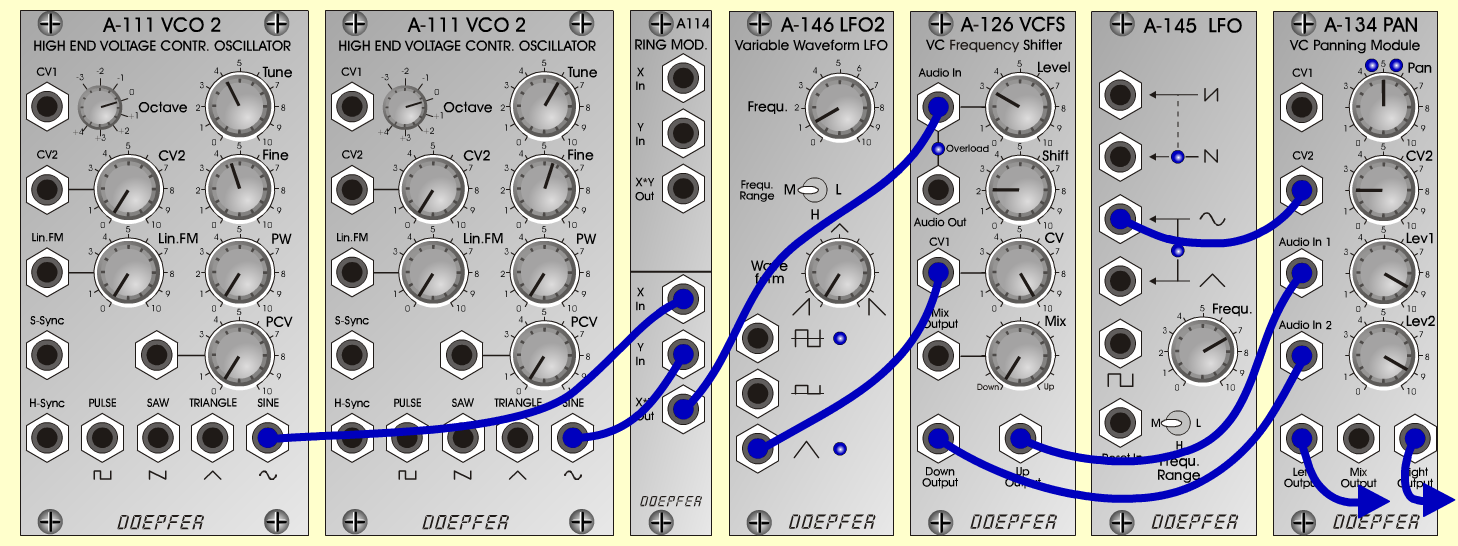Flying SaucerFXD o e p f e r A - 100 Connections: Settings: A-111/1 (Sine) <=> A-114 (X In) A-111/2 (Sine) <=> A-114 (Y In) A-114 (Out) <=> A-126 (Audio In) A-146 (Triangle) <=> A-126 (CV 1) A-126 (Down) <=> A-134 (Audio In 1) A-126 (Up) <=> A-134 (Audio In 2) A-145 (Sine) <=> A-134 (CV 2) A-134 (Left) <=> Amplifier (Left) A-134 (Right) <=> Amplifier (Right) A-111/1 (Octave = 0, Tune = 4.1, Fine = 4.4) A-111/2 (Octave = 0, Tune = 6, Fine = 5.6) A-146 (Freq = 1, Wave Form = 0, Range = M) A-126 (Level = 3, Shift = 2, CV = 10) A-145 (Frq = 7, Range = M) A-134 (Pan = 5, CV 2 = 2, Lev 1 = 9, Lev 2 = 9) Josef MuellerSound samples Flyingsaucer 1 (up) Flyingsaucer 2 (up + reverb) Flyingsaucer 3 (down, A-146 Waveform = 10) Sound samples with reverb: [played with A-198 (CV) <=> A-126 (CV1)] Flyingsaucer 4 Flyingsaucer 5 Flyingsaucer 6 Flyingsaucer 7